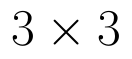Select Page

# Vector Algebra MCQ CBSE Maths 12 Science Answers in English

Vector Algebra MCQ CBSE Maths 12 Science Answers in English to enable students to get Answers in a narrative video format for the specific question.

Expert Teacher provides Vector Algebra MCQ CBSE Maths 12 Science Answers through Video Answers in English language. This video solution will be useful for students to understand how to write an answer in exam in order to score more marks. This teacher uses a narrative style for a question from Vector Algebra not only to explain the proper method of answering question, but deriving right answer too.

Please find the question below and view the Answer in a narrative video format.

Question:

Answer Video in English:

You can select video Answers from other languages also. Please check Answers in ( Hindi )

## Similar Questions from CBSE, 12th Science, Maths, Vector Algebra

Question 1 : Find the angle between the vectorsand(View Answer Video)

Question 2 : Write the position vector of the point which divides the join of points with position vectorsandin the ratio 2:1.  (View Answer Video)

Question 3 : Find |a| and |b|, if (a + b).(a - b) = 8 and |a| = 8|b|. (View Answer Video)

Question 4 :  Find the direction cosines of the vector joining the points A(1, 2, -3) and B(-1, -2, 1) directed from B to A. (View Answer Video)

Question 5 : Find the scalar and vector components of the vector with initial point (2, 1) and terminal point (-5, 7). (View Answer Video)

### Probability

Question 1 :  Bag I contains 3 red and 4 black balls and Bag II contains 4 red and 5 black balls. Two balls are transferred at random from Bag I to Bag II and then a ball is drawn from Bag II.  The ball so drawn is found to be red in colour. Find the probability that the transferred balls were both black.   (View Answer Video)

Question 2 :  A die is rolled. If E= (1, 3, 5), F(2, 3) and G= {2, 3, 4, 5}, find(View Answer Video)

Question 3 : If the mean and variance of a Binomial distribution are 9 and 6 respectively, find the number of trails. (View Answer Video)

Question 4 : Ifandfindif E and F are independent events.  (View Answer Video)

Question 5 : Find the mean, the variance and the standard deviation of the number of doublets in three throws of a pair of dice.  (View Answer Video)

### Determinant

Question 1 : Evaluate. (View Answer Video)

Question 2 : Which of the following is correct? (View Answer Video)

Question 3 : Let A be the non- singular square matrix of order, then |adj A| is equal to, (View Answer Video)

Question 4 : If A is an invertible matrix of order 2, thenis equal to, (View Answer Video)

Question 5 : If a, b, c are in AP, then determinant. (View Answer Video)

### Relations and Functions

Question 1 : Let A = {1, 2, 3}. Then, number of equivalence relations containing (1, 2) are, (View Answer Video)

Question 2 : Ifis defined by, write f(f(x)). (View Answer Video)

Question 3 :  A functionis surjective if and only if , (View Answer Video)

Question 4 : Number of binary sets on the setis, (View Answer Video)

Question 5 : A functionis bijective if and only if, (View Answer Video)